Notes on Acceleration and Retardation | Grade 8 > Science > Velocity and Acceleration | KULLABS.COM

• Note
• Things to remember
• Videos
• Exercise
• Quiz

#### AccelerationAcceleration is defined as the change in velocity per unit time. Acceleration is measured in m/s2.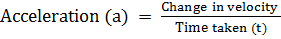=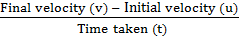Retardation

The negative acceleration is called retardation or deceleration. Its unit is same as acceleration.

Example:

1. Car starts from rest. After 5 seconds, its velocity becomes 10 m/s. Then find its acceleration.

Initial velocity (u) = 0

Final velocity (v) = 10 m/s

Time taken (t) = 5 s

Acceleration (a) =?

Or,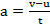Or,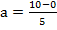∴ a = 2m/s2

2. The Car which starts from the rest has an acceleration of 0.3 m/s2. After 1 minute what will be its acceleration? What is the distance travelled by car?

Initial velocity (u) = 0

Time taken (t) = 1 min= 1 × 60 s = 60 s

Acceleration (a) = 0.3 m/s2

Final velocity (v) =?

Distanced travelled (s) =?

According to formula, v= u+ at

Or, v = 0 + 0.3 × 60

V= 18 m/s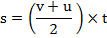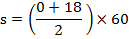∴ s =540 m

Equation of motion of uniform accelerationEquations involving displacement, initial velocity, final velocity, acceleration and time of the motion of a moving body are equations of motion.

Considering a body moving in a straight line with uniform acceleration is shown in the figure.

Let,

Displacement = s

Initial velocity = u

Final velocity = v

Acceleration = a

Time taken = t

Relation between u, v, a and t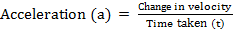Or,Or, at = v-u

∴ v= u + at ………….. (i)

This is the first equation of motion.

Relation between u,s, v and t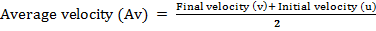Or,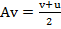Or, $$\text{Average Velocity}=\frac{\text{Total Displacement(s)}}{\text{Total Time Taken(t)}}$$

Or,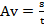As both, equation is equal

Or,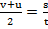Or, 2s = (u + v)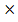t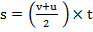……………… (ii)

This is the second equation of the motion.

Relation between s, u, a and t

V= u + at………….. (i)

s = (u + v)t…………… (ii)

Putting value of v from equation (i) in (ii)

Or,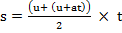Or, s =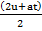Or, s = 2u×t + at × t× ½

∴ s = ut + ½ at2………………. (iii)

This is the third equation of motion.

• Acceleration is defined as the change in velocity per unit time. Acceleration is measured in m/s2.
• The negative acceleration is called retardation or deceleration. Its unit is same as acceleration.
• Equations involving displacement, initial velocity, final velocity, acceleration and time of motion of a moving body are equations of motion.

.

#### Click on the questions below to reveal the answers

Acceleration is defined as the change in velocity per unit time. Acceleration is measured in m/s2.

Solution

Initial velocity (u) = 0

Final velocity (v) = 10 m/s

Time taken (t) = 5 s

Acceleration (a) =?

Or,Or,∴ a = 2m/s2

Solution:

Initial velocity (u) = 0

Time taken (t) = 1 min= 1 × 60 s = 60 s

Acceleration (a) = 0.3 m/s2

Final velocity (v) =?

Distanced travelled (s) =?

According to formula, v= u+ at

Or, v = 0 + 0.3 × 60

V= 18 m/s∴ s =540 m

Solution:Or,Or, at = v-u

∴ v= u + at ………….. (i)

This is the first equation of motion.Or,Or,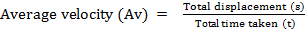Or,As both, equation is equal

Or,Or, 2s = (u + v)t……………… (ii)

This is the second equation of the motion.

Solution:

V= u + at………….. (i)

s = (u + v)t…………… (ii)

Putting value of v from equation (i) in (ii)

Or,Or, s =Or, s = 2u×t + at × t× ½

∴ s = ut + ½ at2………………. (iii)

This is the third equation of motion.

0%

retardation

reduction

induction

deduction

• ### Velocity of car cannot be changed by ______.

Changing the direction

changing the speed

changing speed and direction

Keeping speed and direction constant

1st law

3rd law

4th law

2nd law

3rd law
1st law
2nd law
4th law

4th law
3rd law
2nd law
1st law

## ASK ANY QUESTION ON Acceleration and Retardation

Forum Time Replies Report
##### Dinesh

A car starts with an velocity of 72 km/hr and stops after 4sec' how far does the car move?